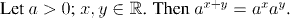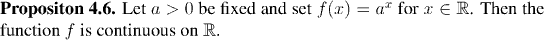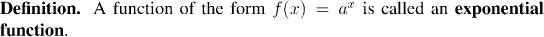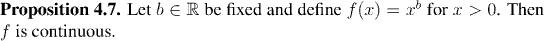Analysis WebNotes
arrow_back arrow_forward

# Class Contents

## Exponential Functions

### Introduction

Given a positive real number a and a real number s, we want to define the quantity a^s. This definition has to extend the understanding we already have of the expression a^s for certain values of s. In particular, we already have definitions for a^s when s is an integer, or the reciprocal of a natural number. The following table summarizes our definitions so far: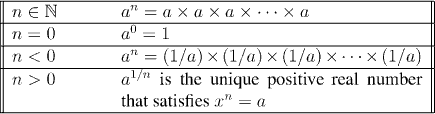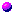Factoid 1:

Exponentiation by an integer exponent has the following properties: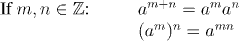The first step in defining a^s for a real exponent, is to define it for a rational exponent. This is our next definition: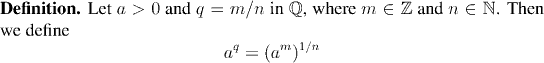Worry:

The problem with this definition is that the same rational q can be expressed in many different ways. Or, put another way, the quotients m/n and k/l may represent the same rational, although m and k, and n and l, are not equal.

We need to show that in this case the rules we follow to obtain a^q using m/n give the same result as when we use k/l.

This type of problem crops up quite frequently in pure mathematics. It is called the problem of showing that a^q is well-defined.Factoid 2: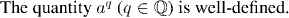Factoid 3: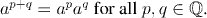Factoid 4: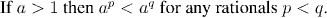We are finally in a position to define the general operation of exponentiation: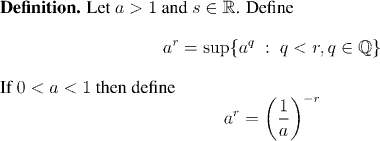Remark:

As before, we have some things to check in order to make sure that this definition makes sense. Unlike our previous definition, however, there is no ambiguity about the exponent. Our worries now are:

1. We need to know that the supremum exists in the real line, and
2. If r is rational, then a^r has already been defined. We need to check that the two definitions are not in conflict.
In view of these two worries, we will hold the definition of a^r in abeyance until after the next two factoids have been proved. Whenever we write a^p in the next two factoids, we shall still be meaning the quantity defined by our first definition, for rational numbers.Factoid 5: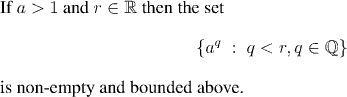Again we emphasize that, for the purposes of the following factoid, a^p has its original meaning from our first definition.Factoid 6: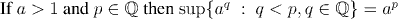Remark: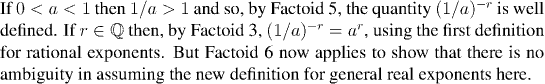From here on, we will always assume that the definition for general real exponents is being used. However, we will also freely use its "backward compatibilty" with the previous definitions (rational or integer exponents) when called for.Factoid 7: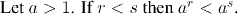Factoid 8: